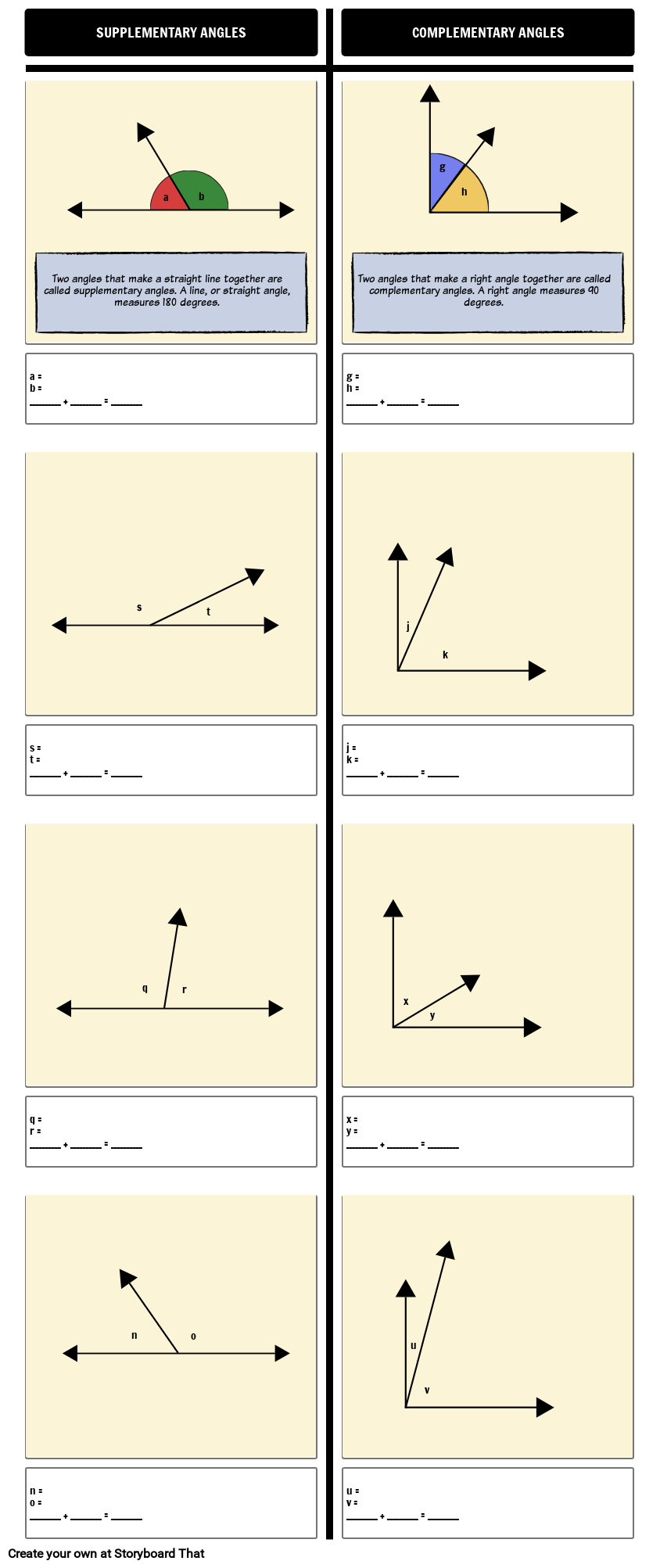## Activity Overview

In this activity, students will measure angles that supplementary or complementary. They are already given the measurement of the large angle (90° or 180°), and they need to find the values of the smaller angle measures. Hopefully, some students will recognize that they really only need to measure one of the angles and then subtract from the whole - or maybe you will explicitly teach that to your students. Discuss efficiency, double-checking, and problem-solving with your students to help their minds prepare to face new challenges with the knowledge they already know.

#### Supplementary Angles

Two angles that make a straight line together are called supplementary angles. A line, or straight angle, measures 180 degrees.

#### Complementary Angles

Two angles that make a right angle together are called complementary angles. A right angle measures 90 degrees.

For some students, using a protractor is extremely difficult, so they may need additional practice, modified assignments, or, most likely, both. Another way to tackle angle measures as additive is to use equations, such as 36° + h = 124°, with or without drawings. With only diagrams of angles, students can figure out how to set up an equation on their own. Without drawings, students strictly use algebra to find the value of the unknown variable rather than using a protractor to find the angle measurements. Both skills in algebra and tool manipulation are important and neither should be neglected.

## Template and Class Instructions

(These instructions are completely customizable. After clicking "Copy Activity", update the instructions on the Edit Tab of the assignment.)

Student Instructions

Determine whether an angle is complementary or supplementary and measure the angles.

1. Click "Start Assignment".
2. Search "Math" or "Protractor" in the search bar and drag down the transparent protractor object.
3. Measure each angle and record the measurements in the description box.#### Lesson Plan Reference

Common Core Standards
• [Math/Content/4/MD/C/7] Recognize angle measure as additive. When an angle is decomposed into non-overlapping parts, the angle measure of the whole is the sum of the angle measures of the parts. Solve addition and subtraction problems to find unknown angles on a diagram in real world and mathematical problems, e.g., by using an equation with a symbol for the unknown angle measure.

## More Storyboard That Activities

### Introducing Angles

*(This Will Start a 2-Week Free Trial - No Credit Card Needed)